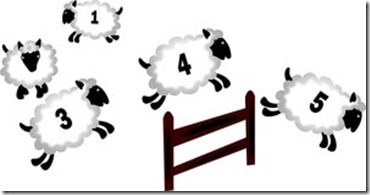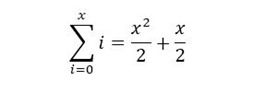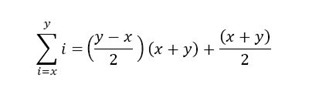# Counting Sheep

Well, what do you think about when you sleep?Many years ago, someone told me how to sum numbers from 0 to 100.  Essentially, just count by 100’s.  0 and 100=100, 1 and 99 = 100, 2 and 98 = 100, etc.  So, there 50 100’s, right?  0-49, plus the 50 left in the middle.  So 50 x 100 = 5,000, plus the 50 stuck in the middle = 5,050.

Easily done.  0 and 200?  100 x 200 = 20,000 plus the 100 in the middle = 20,100.  Keep the series going and you get 45,150, 80,200, etc.

So, I can’t sleep.  Thinking it through is fine, but it would be pretty cool to have a formula!  Not useful, but… it’s something to think about.  So, converting how I explained it above to more mathematical terms, I take 100, divide by 2, then multiple it by 100.  And don’t forget to add the stray 50 in the middle.  That makes sense, but you can’t really have a formula without a variable, so… I’m essentially taking half of x, multiplying it by x, and then adding the change, which is x divided by two.   So x/2 (x) + x/2.  More properly:… where x = the upper limit of the series, which begins at 0.

So, 100 x 100 = 10,000.  Divide by two and add the change = 5,050.  It works, and I cede to sleep, my work completed.

It laid there a while, satisfied.  I go to sleep pretty quickly, so it was months later before I gave it another thought, expecting I’d have to move on to some non-mathematical thing before falling asleep.   Yeah.  But, wait…   There’s still the utility of the thing.   Where would I find that, exactly?  Well, back in grade school, I guess.  Math class.  The teacher asks for the sum for extra credit, and I can quickly do that.  They want a formula for it?  Ha.  I’m ahead of you on that one.  And then there’s another quiz, we’ll say, for summing numbers between, oh, 60 and 170, and well, I haven’t actually tried that

Those numbers are too much to deal with, so… I can handle a range of 10 to 20 without a calculator while noodling over this.  One might think, and hope, that just adding those particular sheep in my head would put me to sleep.  It didn’t.  The answer is 165.

Now, admittedly, I didn’t actually add them.  Counting from 10 to 20, I have 11 base values of 10, and I can use my little formula for the change from each number (0 from 10, 1 from 11, 2 from 12… 10 from 20).  So, 11 x 10 = 110 from the base value, then my little formula works for the 0 to 10 of the leftover digits:  102/2 = 50.  Add the 5 left over, and we get 55.  110 + 55 = 165.  Maybe I should have just used 2- 7 for a proof?

Anyway, following my original formula, 202/2 + 10 = 210.   Not good.  My little formula is no good for anything other than a very specific parlor trick.

So, let’s backtrack and see what can work.  I need another variable, resetting to:

• x = lower number
• y= higher number

The numbers in the series no longer add to the upper number in the range, unless the range starts with zero, but the same “easy math” matching process is the same – 10 and 20 = 30, 11 and 19 = 30, 12 and 18 = 30, etc.  So, there’s a strong similarity to what I was doing before as that is the number I’m multiplying against, x + y.  Now I need to figure out how to express how many times I’m multiplying against it.

From 0 to 100, the midpoint happens to be the number of times to use, but that doesn’t apply in the range of 10 to 20, because there’s only 11 number being summed.  How many counts are there before I reach the midpoint?  Well, the midpoint is 15, so… 5.  So, it’s the difference between the two numbers, divided by two (y – x)/2, not the average (y + x)/2 which was implied when I started at zero in the original formula.

So, the number of times is (y-x)/2.  Multiplied against the sum (x+y), then adding the change, which is now more properly described as half of the sum rather than the number in the middle of the range (x+y)/2.  Let’s test that.

• 20 – 10 = 10.
• 10/2 = 5.
• 5 x (10 + 20) = 150.
• Plus the change, 30/2 = the 15 left in the middle.
• 150 + 15 = 165.

Bingo.  And no bothersome “squaring” a number, either.

For not-yet-asleep fun, back to my original problem of 0 to 100:

• 100 – 0 = 100
• 100/2 = 50
• 50 x 100 = 5,000
• Plus the change, the 50 left in the middle = 5,050.1.